# RS Aggarwal Solutions for Class 6 Maths Chapter 17 Quadrilaterals

RS Aggarwal Solutions for Class 6 Maths Chapter 17 Quadrilaterals are accessed here. Students can go through RS Aggarwal Solutions for Class 6 Chapter 17 to understand and clear their doubts about Quadrilaterals. By regularly practicing RS Aggarwal textbook questions, students will become experts in Mathematics along with scoring high marks in examination. Those who want to strengthen their concepts in Quadrilaterals are advised to go through RS Aggarwal Solutions for Class 6 Chapter 17.

RS Aggarwal Solutions are available in PDF format which have been solved by experts at BYJU’S. Download PDF of Class 6 Chapter 17 in their respective links.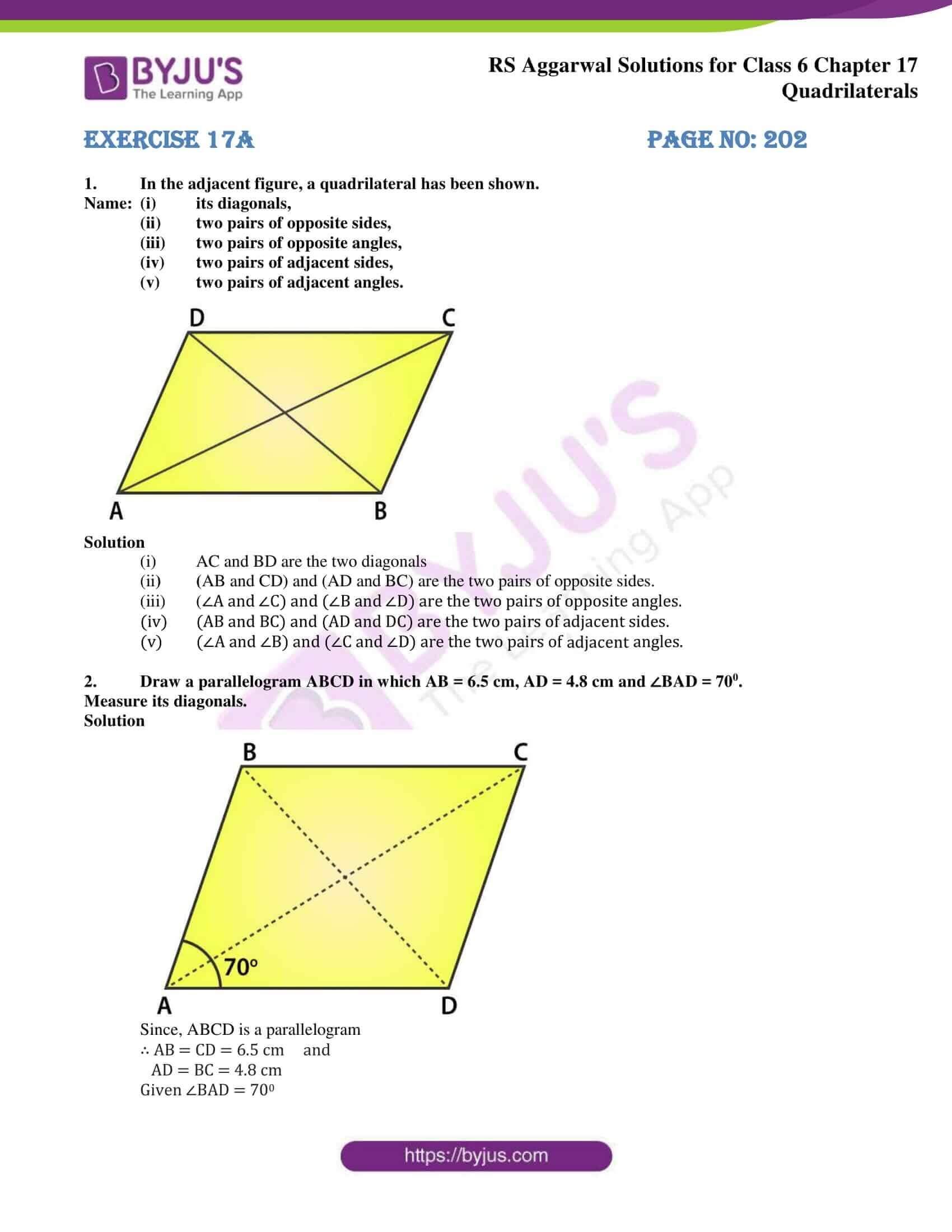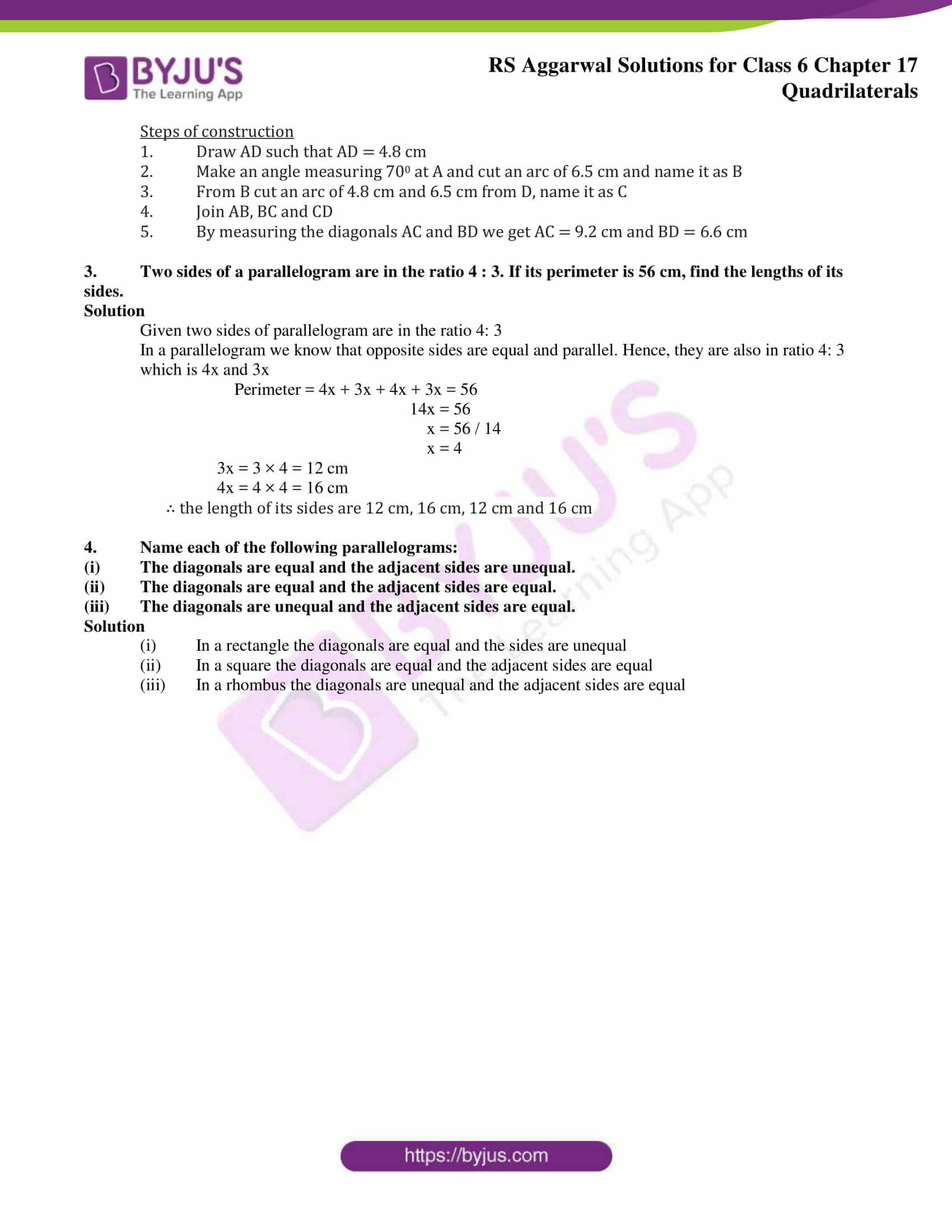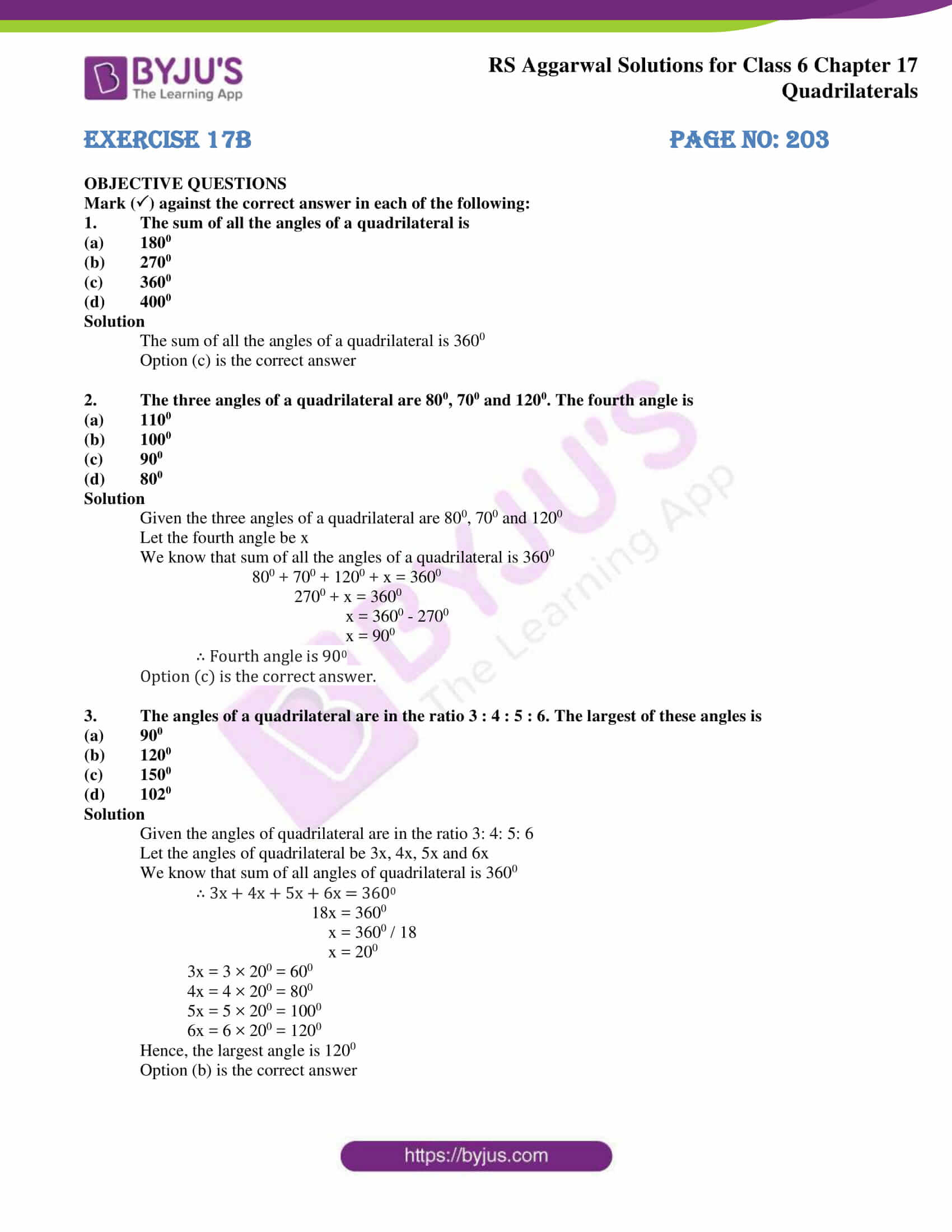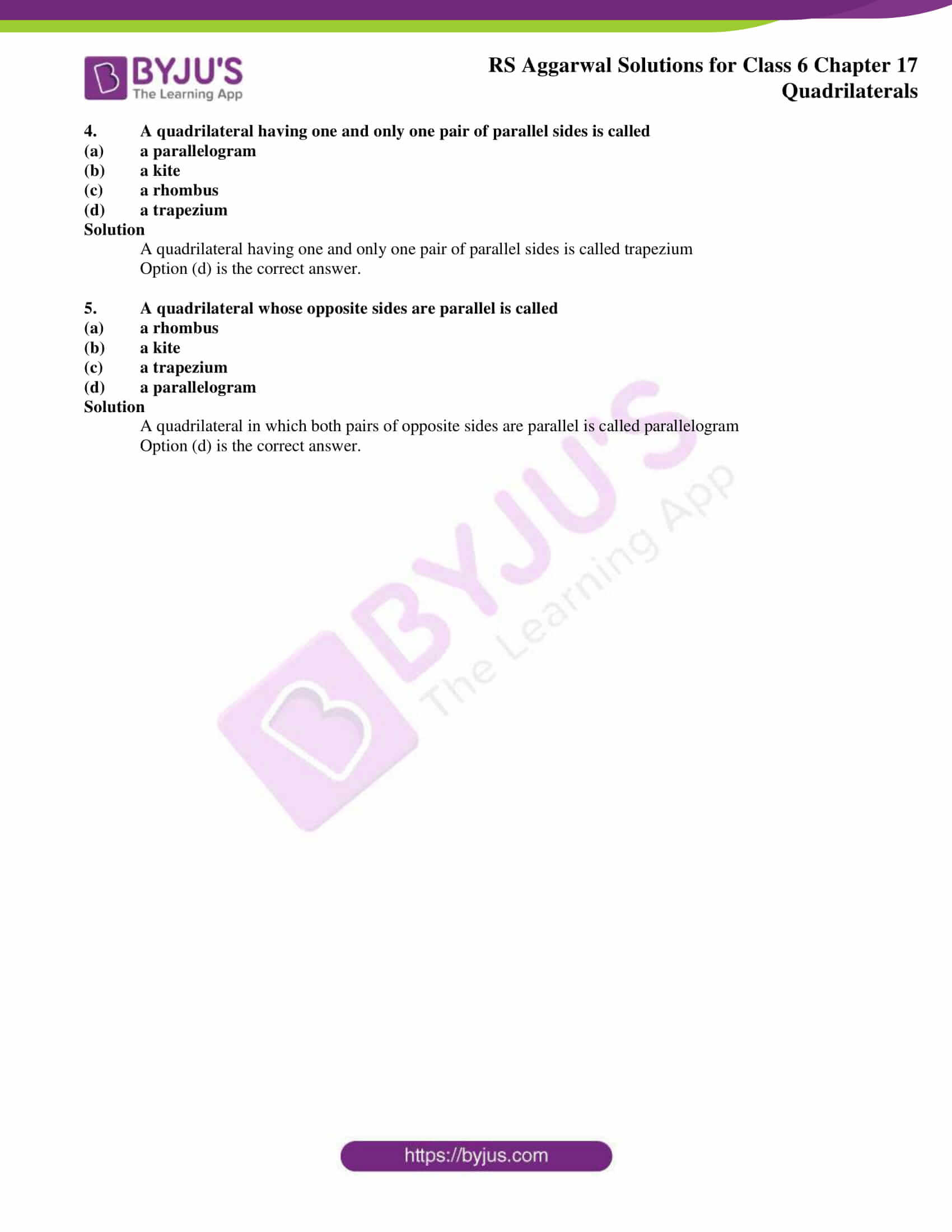### Access answers of RS Aggarwal Solutions for Class 6 Maths Chapter 17 – Quadrilaterals

Exercise 17A PAGE NO: 202

Name: (i) its diagonals,

(ii) two pairs of opposite sides,

(iii) two pairs of opposite angles,

(iv) two pairs of adjacent sides,

(v) two pairs of adjacent angles.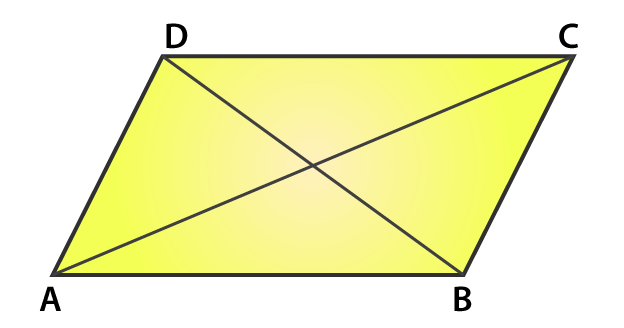Solution

(i) AC and BD are the two diagonals

(ii) (AB and CD) and (AD and BC) are the two pairs of opposite sides.

(iii) (∠A and ∠C) and (∠B and ∠D) are the two pairs of opposite angles.

(iv) (AB and BC) and (AD and DC) are the two pairs of adjacent sides.

(v) (∠A and ∠B) and (∠C and ∠D) are the two pairs of adjacent angles.

2. Draw a parallelogram ABCD in which AB = 6.5 cm, AD = 4.8 cm and ∠BAD = 700.

Measure its diagonals.

Solution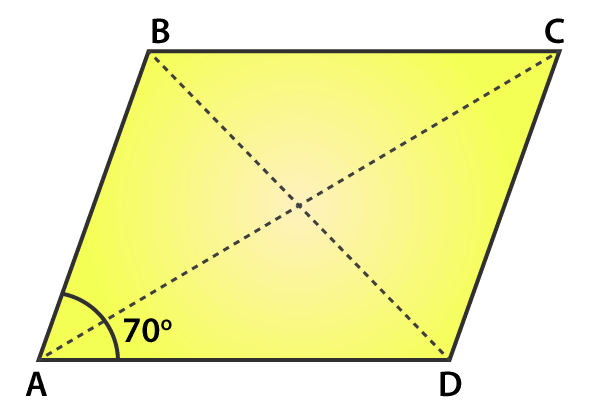Since, ABCD is a parallelogram

∴ AB = CD = 6.5 cm and

AD = BC = 4.8 cm

Steps of construction

2. Make an angle measuring 700 at A and cut an arc of 6.5 cm and name it as B

3. From B cut an arc of 4.8 cm and 6.5 cm from D, name it as C

4. Join AB, BC and CD

5. By measuring the diagonals AC and BD we get AC = 9.2 cm and BD = 6.6 cm

3. Two sides of a parallelogram are in the ratio 4 : 3. If its perimeter is 56 cm, find the lengths of its sides.

Solution

Given two sides of parallelogram are in the ratio 4: 3

In a parallelogram we know that opposite sides are equal and parallel. Hence, they are also in ratio 4: 3 which is 4x and 3x

Perimeter = 4x + 3x + 4x + 3x = 56

14x = 56

x = 56 / 14

x = 4

3x = 3 × 4 = 12 cm

4x = 4 × 4 = 16 cm

∴ the length of its sides are 12 cm, 16 cm, 12 cm and 16 cm

4. Name each of the following parallelograms:

(i) The diagonals are equal and the adjacent sides are unequal.

(ii) The diagonals are equal and the adjacent sides are equal.

(iii) The diagonals are unequal and the adjacent sides are equal.

Solution

(i) In a rectangle the diagonals are equal and the sides are unequal

(ii) In a square the diagonals are equal and the adjacent sides are equal

(iii) In a rhombus the diagonals are unequal and the adjacent sides are equal

Exercise 17B PAGE nO: 203

OBJECTIVE QUESTIONS

Mark () against the correct answer in each of the following:

1. The sum of all the angles of a quadrilateral is

(a) 1800

(b) 2700

(c) 3600

(d) 4000

Solution

The sum of all the angles of a quadrilateral is 3600

Option (c) is the correct answer

2. The three angles of a quadrilateral are 800, 700 and 1200. The fourth angle is

(a) 1100

(b) 1000

(c) 900

(d) 800

Solution

Given the three angles of a quadrilateral are 800, 700 and 1200

Let the fourth angle be x

We know that sum of all the angles of a quadrilateral is 3600

800 + 700 + 1200 + x = 3600

2700 + x = 3600

x = 3600 – 2700

x = 900

∴ Fourth angle is 900

Option (c) is the correct answer.

3. The angles of a quadrilateral are in the ratio 3 : 4 : 5 : 6. The largest of these angles is

(a) 900

(b) 1200

(c) 1500

(d) 1020

Solution

Given the angles of quadrilateral are in the ratio 3: 4: 5: 6

Let the angles of quadrilateral be 3x, 4x, 5x and 6x

We know that sum of all angles of quadrilateral is 3600

∴ 3x + 4x + 5x + 6x = 3600

18x = 3600

x = 3600 / 18

x = 200

3x = 3 × 200 = 600

4x = 4 × 200 = 800

5x = 5 × 200 = 1000

6x = 6 × 200 = 1200

Hence, the largest angle is 1200

Option (b) is the correct answer

4. A quadrilateral having one and only one pair of parallel sides is called

(a) a parallelogram

(b) a kite

(c) a rhombus

(d) a trapezium

Solution

A quadrilateral having one and only one pair of parallel sides is called trapezium

Option (d) is the correct answer.

5. A quadrilateral whose opposite sides are parallel is called

(a) a rhombus

(b) a kite

(c) a trapezium

(d) a parallelogram

Solution

A quadrilateral in which both pairs of opposite sides are parallel is called parallelogram

Option (d) is the correct answer.

### RS Aggarwal Solutions for Class 6 Maths Chapter 17 Quadrilaterals

Chapter 17 – Quadrilaterals contain 2 exercises.Each question is solved in such a way that students are able to understand them easily. Let us go through the topics which are discussed in this chapter.

• Interior and exterior of a Quadrilateral
• Angle sum property of a Quadrilateral
• Properties of a parallelogram
• Properties of a rhombus
• Properties of a rectangle
• Properties of a square

Also, access RS Aggarwal Solutions for Class 6 Chapter 17 Exercises

Exercise 17A

Exercise 17B

### Chapter Brief of RS Aggarwal Solutions for Class 6 Maths Chapter 17 – Quadrilaterals

A closed figure or a polygon with four sides and four angles is known as a Quadrilateral. Parallelogram, rectangle, square, trapezoid and rhombus are the different types of Quadrilaterals. Mobiles, laptops, computers, books, copies, chart papers etc. are examples of Quadrilaterals.